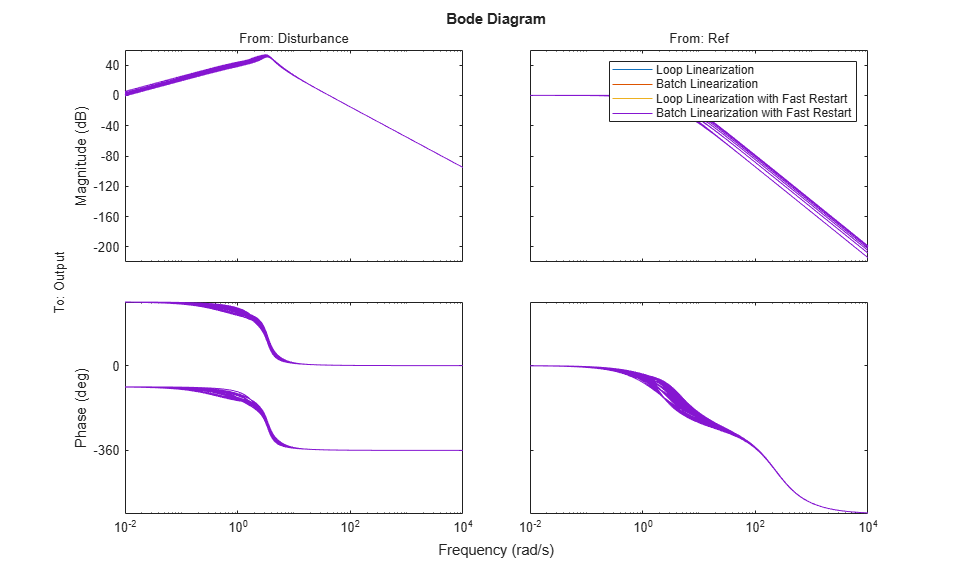Improve Linear Analysis Performance

This example shows how to use the fastRestartForLinearAnalysis function to speed up multiple calls to compiling functions in Simulink® Control Design™ software such as findop and linearize.

Run Linear Analysis Commands in a Loop

In this example, you trim and linearize a closed-loop engine speed control model. You vary the PI control parameters and observe how the closed-loop behavior changes at steady state. Since linearize and findop are called in a loop, the model compiles 2*N + 1 times including the first call to operspec.

Open the engine speed control model and obtain the linear analysis points from the model.

mdl = 'scdspeedctrl';
open_system(mdl)
io = getlinio(mdl);
fopt = findopOptions('DisplayReport','off');Configure the PI controller to use the base workspace variables kp and ki.

blk = [mdl,'/PID Controller'];
set_param(blk,'P','kp');
set_param(blk,'I','ki');

Create a grid of parameter values to vary.

vp = 0.0005:0.0005:0.003;
vi = 0.0025:0.0005:0.005;
[KP,KI] = ndgrid(vp,vi);
N = numel(KP);
sz = size(KP);

Initialize the base workspace variables.

kp = KP(1);
ki = KI(1);

Run the loop and record execution time.

t = cputime;
ops = operspec(mdl);
for i = N:-1:1
kp = KP(i);
ki = KI(i);
% Trim the model.
op = findop(mdl,ops,fopt);
[j,k] = ind2sub(sz,i);
% Linearize the model.
sysLoop(:,:,j,k) = linearize(mdl,io,op);
end

Calculate the elapsed time.

timeElapsedLoop = cputime - t;

Run Linear Analysis Commands in Batch

Rather than loop over the parameters, findop and linearize can accept a batch parameter variation structure directly to reduce the number of times the model compiles. In this case, the model compiles three times with calls to operspec, findop, and linearize.

Run and record execution time.

t = cputime;
ops = operspec(mdl);

Create the batch parameter structure.

params(1).Name  = 'kp';
params(1).Value =  KP ;
params(2).Name  = 'ki';
params(2).Value =  KI ;

Trim the model across the parameter set.

op = findop(mdl,ops,params,fopt);

Linearize the model across the parameter and operating point set.

sysBatch = linearize(mdl,io,op,params);

Calculate the elapsed time.

timeElapsedBatch = cputime - t;

Run Linear Analysis Functions in Loop with Fast Restart

The fastRestartForLinearAnalysis function configures the model to minimize compilations even when compiling functions are run inside a loop. The model compiles with calls to operspec, findop, and linearize in a loop.

Run the loop and record execution time with fast restart for linear analysis enabled.

t = cputime;

Enable fast restart for linear analysis. Provide linear analysis points to minimize compilations between calls to findop and linearize.

fastRestartForLinearAnalysis(mdl,'on','AnalysisPoints',io);

Trim and linearize the model in a loop.

ops = operspec(mdl);
for i = N:-1:1
kp = KP(i);
ki = KI(i);
% Trim the model.
op = findop(mdl,ops,fopt);
[j,k] = ind2sub(sz,i);
% Linearize the model.
sysFastRestartLoop(:,:,j,k) = linearize(mdl,io,op);
end

Turn off fast restart for linear analysis, which uncompiles the model.

fastRestartForLinearAnalysis(mdl,'off');

Calculate the elapsed time.

timeElapsedFastRestartLoop = cputime - t;

Run Linear Analysis Functions in Batch with Fast Restart

You can further improve performance by enabling fast restart for linear analysis and executing the batch linearize and findop functions. In this case, the model compiles once with calls to operspec, findop, and linearize.

Run and record execution time with fast restart for linear analysis on.

t = cputime;

Enable fast restart for linear analysis. Provide linear analysis points to minimize compilations between calls to findop and linearize.

fastRestartForLinearAnalysis(mdl,'on','AnalysisPoints',io)

Create the batch parameter structure.

params(1).Name  = 'kp';
params(1).Value =  KP ;
params(2).Name  = 'ki';
params(2).Value =  KI ;

Trim the model across the parameter set.

ops = operspec(mdl);
op = findop(mdl,ops,params,fopt);

Linearize the model across the parameter and operating point set.

sysFastRestartBatch = linearize(mdl,io,op,params);

Disable fast restart for linear analysis, which uncompiles the model.

fastRestartForLinearAnalysis(mdl,'off');

Calculate the elapsed time.

timeElapsedFastRestartBatch = cputime - t;

Compare the Results

Compare the linearization results of the four methods. The bode plot below shows that each method returns the same results.

compareIdx = 1:N;
bode(...
sysLoop(:,:,compareIdx), ...
sysBatch(:,:,compareIdx), ...
sysFastRestartLoop(:,:,compareIdx), ...
sysFastRestartBatch(:,:,compareIdx));
legend(...
'Loop Linearization', ...
'Batch Linearization', ...
'Loop Linearization with Fast Restart', ...
'Batch Linearization with Fast Restart')Compile the elapsed time and speed-up ratio for each method in a table. You can obtain significant performance gains using batch trimming and linearization as well as fastRestartForLinearAnalysis.

Method = ["Loop","Batch","Fast Restart Loop","Fast Restart Batch"]';
TimeElapsed = [timeElapsedLoop timeElapsedBatch ...
timeElapsedFastRestartLoop timeElapsedFastRestartBatch]';
SpeedUpFactor = TimeElapsed(1)./TimeElapsed;
TimeElapsedTable = table(Method,TimeElapsed,SpeedUpFactor)
TimeElapsedTable =

4x3 table

Method           TimeElapsed    SpeedUpFactor
____________________    ___________    _____________

"Loop"                    122.54               1
"Batch"                    39.49          3.1031
"Fast Restart Loop"        52.35          2.3408
"Fast Restart Batch"       35.82           3.421